# CLASS 9 MATH NCERT SOLUTION FOR CHAPTER – 9 AREAS OF PARALLELOGRAMS AND TRIANGLES EX – 9.3

## Areas of Parallelograms and Triangles

Question 1.
In the given figure, E is any point on median AD  of a Δ ABC. Show that
ar (Δ ABE) = ar(Δ ACE).

Solution.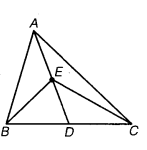Given: AD is a median of Δ ABC and E is any point on AD.
To prove: ar (Δ ABE) – ar (Δ ACE)
Proof: Since AD is the median of Δ ABC.
∴ ar(Δ ABD) = ar (Δ ACD)    …(i)
[since a median of a triangle divides it into two triangles of equal areas]
Also, ED is the median of AF.RC
∴ ar (ABED) = ar (ACED)      … (ii)
[since a median of a triangle divides it into two triangles of equal areas] On subtracting eq. (ii) from eq. (i), we get
ar(Δ ABD) – ar (Δ BED) = ar (Δ ACD) – ar (ΔCED)
⇒  ar (Δ ABE) = ar (Δ ACE)
Hence proved

Question 2.
In a AABC, E is the mid-point of median AD. Show that
ar(ΔBED) =$\frac { 1 }{ 4 }$ ar (Δ ABC).

Solution.Given: ABC is a triangle and E is the mid-point of the median AD.
To prove :     ar (Δ BED) =$\frac { 1 }{ 4 }$ ar (Δ ABC)
Proof : We know that the median divides a triangle into two triangles of equal areas.
∴ ar (Δ ABD) = ar(Δ  ADC)
⇒  ar (Δ ABD) =$\frac { 1 }{ 2 }$ ar (Δ ABC)  …(i)
In Δ ABD, BE is the median.
ar (ΔBED) = ar (ΔBAE)
⇒  ar(ΔBED) =$\frac { 1 }{ 2 }$ar (ΔABD)
⇒ ar(ΔBED) =$\frac { 1 }{ 2 }$.$\frac { 1 }{ 2 }$ ar (ΔABC)
[from eq. (i)]
⇒ ar(ΔBED) =$\frac { 1 }{ 4 }$ ar (ΔABC)
Hence proved.

Question 3.
Show that the diagonals of a parallelogram divide it into four triangles of equal area.

Solution.Given: ABCD is a parallelogram and its diagonals AC and BD intersect each other at O.
To prove: Diagonals AC and BD divide parallelogram ABCD into four triangles of equal areas.
i.e., ar (Δ OAB) = ar (Δ OBC) = ar (Δ OCD) = ar (Δ OAD)
Proof: We know that the diagonals of a parallelogram bisect each other, so we have
OA = OC and OB = OD.
Also, we know that a median of a triangle divides it into two triangles of equal areas.
Now, as in Δ ABC, BO is the median.
∴ ar (Δ OAB) = ar (Δ OBC)  … (i)
In Δ ABD, AO is the median.
∴  ar (Δ OAB) = ar (Δ OAD) … (ii)
Similarly, in Δ ACD, DO is the median.
ar (Δ AOD) = ar (Δ OCD)     …(iii)
From eqs. (i), (ii) and (iii), we get
ar (Δ OAB) = ar (Δ OBC) = ar (ΔOCD) = ar (Δ OAD) lienee proved.

Question 4.
In the given figure, ABC and ABD are two triangles on the same base AB. If the line-segment CD is bisected by AB at O, show that ar(Δ ABC )= ar( Δ ABD)

Solution.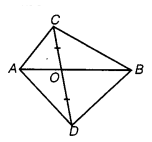Given: ABC and ABD are two triangles on the same base AB.
To prove : ar (ΔABC) = ar (Δ ABD)
Proof: Since the line segment CD is bisected by AB at O.
∴ OC = OD
In Δ ACD, we have OC = OD
So, AO is the median of ΔACD.
Also, we know that the median divides a triangle into two triangles of equal areas.
∴ ar (Δ AOC) = ar (ΔAOD)  … (i)
Similarly, in ABCD,
ar (ABOC) = ar (ABOD)    … (ii)
[Since, BO is the median of ABCD]
On adding eqs. (i) and (ii), we get ar (Δ AOC) + ar (Δ BOC) = ar (Δ AOD) + ar (Δ BOD)
⇒  ar (Δ ABC) = ar (Δ ABD)
Hence proved.

Question 5.
D, E and F are respectively the mid-points of the sides BC, CA and AB of a ΔABC.Show that

(i) BDEF is a parallelogram.
(ii) ar (ΔDEF) =$\frac { 1 }{ 2 }$ ar (ΔABC).
(iii) ar (||gm BDEF) = ar (AABC).

Solution.Given: ABC is a triangle in which the mid-points of sides BC, CA and AB
(i) are respectively D, E, and F.
To prove :
BDEF is a parallelogram.
(ii) ar (ΔDEF) =$\frac { 1 }{ 4 }$ ar (AABC)
(iii) ar (||gm BDEF) =$\frac { 1 }{ 2 }$ ar (AABC)
(i)
Proof:
Since, E and F are the mid-points of AC and AB.
BC || FE and FE =$\frac { 1 }{ 2 }$ BC =BD [by mid-point theorem]
=> BD || FE and BD = FE
Similarly, BF || DE and BF = DE
Hence, BDEF is a parallelogram.
[∵ a pair of opposite sides are equal and parallel]

(ii) Similarly, we can prove that both FDCE and AFDE are also parallelograms.
Now, BDEF is a parallelogram, so its diagonal FD divides it’s into two triangles of equal areas.Question 6.
In the adjoining figure, diagonals AC and BD of quadrilateral ABCD intersect at O such that OB = OD. If AB = CD, then show that

(i) ar (Δ DOC) = ar (Δ AOB).
(ii) ar (Δ DCE) = ar (Δ ACB.)”
(iii) DA || CB or ABCD is a parallelogram.Solution.
Given: ABCD is a quadrilateral in which AB = CD and its diagonals AC and BD intersect at O such that
OB = OD.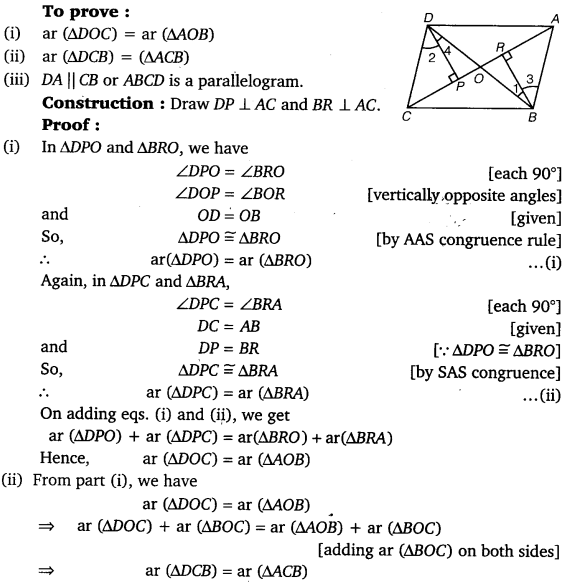Question 7.
D and E are points on sides AB and AC respectively of Δ ABC such that ar (Δ DBO = ar (Δ EBC). Prove that DE || BC.

Solution.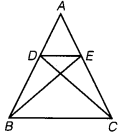Given: A Δ ABC and D and E are points on sides AB and AC respectively, such that
ar (Δ DBC) = ar (Δ EBC).
To prove:   DE || BC
Proof: Here, ADBC and AEBC are equal in area and have same base BC.
∴ Altitude from D of Δ DBC Altitude from E of Δ EBC
Hence, Δ DBC and Δ AEBC are between the same parallels.
i.e., DE || BC

Question 8.
XY is a line parallel to side BC of an AABC. If BE || AC and CF || AB meet XY at E and F respectively, show that ar (Δ ABE) = ar (Δ ACF).

Solution.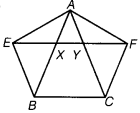Given A Δ ABC in which XY || BC, BE || AC,
i.e. BE || CY and CF || AB, i.e., CF || XB.
To prove :  ar (Δ ABE) = ar (Δ ACF)
Proof: Since, XY || BC and CY || BE. So, EYCB is a parallelogram.
Now, as Δ ABF and parallelogram EYCB lie on the same base BE and CA.
ar (AABE) =$\frac { 1 }{ 2 }$ ar (||gm  BCYE)     …(i)
Again, CF || BX and ZF || BC So, BCFX is a parallelogram.
Now, as Δ ACF and parallelogram BCFX lie on the same base CF and between the same parallel lines AB and FC.
ar (Δ ACF) =$\frac { 1 }{ 2 }$ ar (||gm BCFX)    …(ii)
Also, parallelograms BCFX and BCYE lie. on the same base BC and between the same parallels BC and EF.
ar (||gm BCFX) = ar (||gm BCYE) …(iii)
From eqs. (i), (ii) and (iii), we get
ar (Δ ABE) = ar (Δ ACF)

Question 9.
The side AB of a parallelogram ABCD is produced to any point P. A line through A and parallel to CP meets CB produced at Q and then parallelogram PBQR is completed (see the figure).
Show that ar (||gm ABCD) = ar (||gm PBQR)

[Hint: Join AC and PQ. Now compare ar (Δ ACQ) and ar (Δ APQ).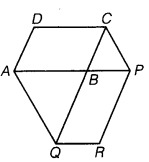Solution.
Given: Two parallelograms ABCD and PBQR.
To prove: ar Q F ABCD) = ar 0 F PBQR)
Construction: Join AC and PQ.
Proof: Since, PQ and AC are diagonals of parallelograms PBQR and ABCD, respectively,Question 10.
Diagonals AC and BD of a trapezium ABCD with AB || DC intersect each other at O. Prove that
ar  (Δ AOD) = ar (Δ BOC).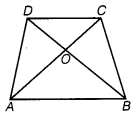Solution.
Given: Diagonals AC and BD of a trapezium ABCD  with AB || DC intersect each other at 0.Question 11.
In the given figure, ABCDE is a pentagon. A line through B parallel to AC meets DC produced at F. Show that
(i) ar (Δ ACB) = ar (Δ ACF).
(ii) ar (||gm  AEDF) = ar (pentagon ABCDE). a b

Solution.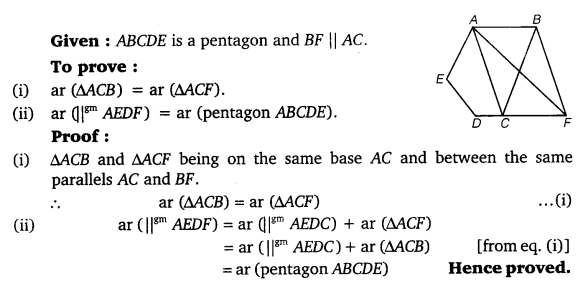Question 12.
A villager Itwaari has a plot of land of the shape of a quadrilateral. The Gram Panchayat of the village decided to take over some portion of his plot from one of the comers to construct a Health Center. Itwaari agrees to the above proposal with the condition that he should be given an equal amount of land in lieu of his land adjoining his plot so as to form a triangular plot. Explain how this proposal will be implemented?

Solution.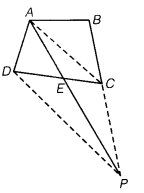Let ABCD be the plot of land in the shape of a quadrilateral. Let the portion ADE be taken over by the Gram Panchayat of the village from one corner D to construct a Health Center. Join AC and draw a line through D parallel to AC to meet BC produced at P. Then, Itwaari must be given the land ECP adjoining his plot so as to form a triangular plot ABP.
Justification

Question 13.
ABCD is a trapezium with AB||DC. A line parallel to AC intersects AB at X and BC at Y. Prove that ar (ΔADX) = ar (Δ ACY). [Hint: Join CX.]

Solution.Question 14.
In figure, AP || BQ || CR. Prove that ar(Δ AQC) = ar(Δ PHR)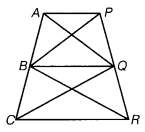Solution.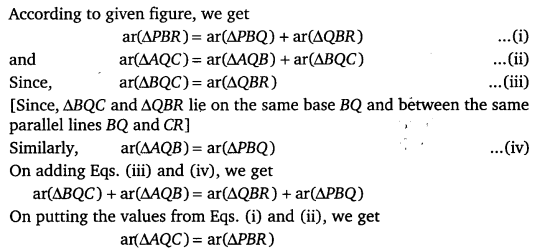Question 15.
Diagonals AC and BD of a quadrilateral ABCD intersect at O in such a way that
ar (Δ AOD) = ar (Δ BOO. Prove that ABCD is a trapezium.

Solution.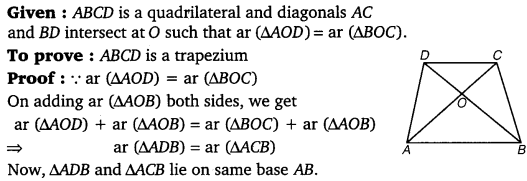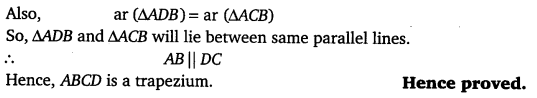Question 16.
In the figure, ar (Δ DRC) = ar (Δ DPC) and ar (Δ BDP) = ai(Δ ARC). Show that both the quadrilaterals ABCD and DCPR are trapeziums.

Solution.#### CLASS 9 MATH NCERT SOLUTION FOR CHAPTER – 9 AREAS OF PARALLELOGRAMS AND TRIANGLES EX – 9.4

Lorem ipsum dolor sit amet, consectetur adipiscing elit. Phasellus cursus rutrum est nec suscipit. Ut et ultrices nisi. Vivamus id nisl ligula. Nulla sed iaculis ipsum.

Company Name